# NEET Solid State Questions : NEET Previous Year Questions

8. The number of carbon atoms per unit cell of diamond unit cell is (NEET 2013)

(a) 6                                 (b) 1

(c) 4                                 (d) 8

Chemistry Notes

Physics Notes

Biology Notes

9. In a face-centred cubic lattice, a unit cell is shared equally by how many unit cells?  (2005)

(a) 2                                 (b) 4

(c) 6                                 (d) 8

13. A compound is formed by cation C and anion A. The anions form hexagonal close packed (hcp) lattice and the cations occupy 75% of octahedral voids. The formula of the compound is                                                    (NEET 2019)

(a) C4A3                         (b) C2A3

(c) C3A2                          (d) C3A4

14. In calcium fluoride, having the fluorite structure, the coordination numbers for calcium ion (Ca2+) and fluoride ion (F) are                       (NEET-II 2016)

(a) 4 and 2                 (b) 6 and 6

(c) 8 and 4                (d) 4 and 8

15. The ionic radii of A+ and B ions are 0.98 × 10–10 m and 1.81 × 10–10 m. The coordination number of each ion in AB is                       (NEET-I 2016)

(a) 8                     (b) 2

(c) 6                     (d) 4

16. The number of octahedral void(s) per atom present in a cubic close-packed structure is  (2012)

(a) 1                                 (b) 3

(c) 2                                 (d) 4

17. Structure of a mixed oxide is cubic close packed  (ccp). The cubic unit cell of mixed oxide is composed  of oxide ions. One fourth of the tetrahedral voids are occupied by divalent metal A and the octahedral             (Mains 2012)

voids are occupied by a monovalent metal B. The formula of the oxide is

(a) ABO2                               (b) A2BO2

(c) A2B3O4                       (d) AB2O2

18. A solid compound XY has NaCl structure. If the radius of the cation is 100 pm, the radius of the anion (Y) will be                                          (Mains 2011)

(a)  275.1 pm           (b) 322.5 pm

(c) 241.5 pm           (d) 165.7 pm

25. An element has a body centered cubic (bcc) structure with a cell edge of 288 pm. The atomic

(a)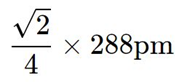(b)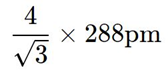(c)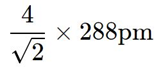(d)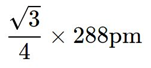26. The vacant space in bcc lattice unit cell is                    (2015, 2008)

(a) 48%                    (b) 23%

(c) 32%                   (d) 26%

27. If a is the length of the side of a cube, the distance between the body-centred atom and one corner atom in the cube will be                            (2014)

(a) 2/√3 a                        (b) 4/√3 a

(c) √3/4 a                         (d) √3/2 a

28. A metal crystallizes with a face-cent red cubic lattice. The edge of the unit cell is 408 pm. The diameter of the metal atom is                                               (2012)

(a) 288 pm              (b) 408 pm

(c) 144 pm             (d) 204 pm

29. AB crystallizes in a body-cent red cubic lattice with edge length ‘a’ equal to 387 pm. The distance between two oppositely charged ions in the lattice is                                 (2010)

(a) 335 pm                          (b) 250 pm

(c) 200 pm                         (d) 300 pm

30. Lithium metal crystallizes in a body-cent red cubic crystal. If the length of the side of the unit cell of lithium is 351 pm, the atomic radius of lithium will be                                  (2009)

(a) 151.8 pm                    (b) 75.5 pm

(c) 300.5 pm                  (d) 240.8 pm

31. Copper crystallizes in a face-cent red cubic lattice with a unit cell length of 361 pm. What is the radius of copper atom in pm?                                     (2009)

(a) 157                      (b) 181

(c) 108                      (d) 128

32. Which of the following statements is not correct?                            (2008)

(a) The number of carbon atoms in a unit cell of diamond is 8.

(b) The number of Brava is lattices in which a crystal can be categorized is 14.

(c) The fraction of the total volume occupied by the atoms in a primitive cell is 0.48.

(d) Molecular solids are generally volatile.

33. If a stands for the edge length of the cubic systems: simple cubic, body-centred cubic and face-centred cubic, then the ratio of radii of the spheres in these systems will be respectively                                                (2008)

(a)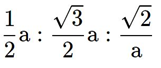(b)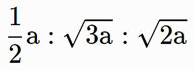(c)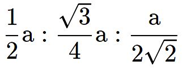(d)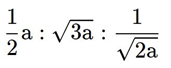34. The fraction of total volume occupied by the atoms present in a simple cube is     (2007)

(a) π / 3√2

(b) π / 4√2

(c) π / 4

(d) π / 6

37. Iron exhibits bcc structure at room temperature. Above 900°C, it transforms to fcc structure. The ratio of density of iron at room temperature to that at 900°C (assuming molar mass and atomic radii of iron remains constant with temperature) is        (NEET 2018)

(a) √3 / √2

(b) 4√3 / 3√2

(c) 3√3 / 4√2

(d) 1 / 2

38. Lithium has a bcc structure. Its density is 530 kg m–3 and its atomic mass is 6.94 g mol–1. Calculate the edge length of a unit cell of lithium metal. (NA = 6.02 × 1023 mol–1)(NEET-I 2016)

(a) 527 pm                    (b) 264 pm

(c) 154 pm                    (d) 352 pm

39. A metal has a fcc lattice. The edge length of the unit cell is 404 pm. The density of the metal is 2.72 g cm–3. The molar mass of the metal is (NEET 2013)

(NA Avogadro’s constant = 6.02 × 1023 mol–1)

(a) 27 g mol–1                (b) 20 g mol–1

(c) 40 g mol–1                      (d) 30 g mol–1

40. CsBr crystallizes in a body-cent red cubic lattice. The unit cell length is 436.6 pm. Given that the atomic mass of Cs = 133 and that of Br = 80 amu and Avogadro number being 6.02 × 1023 mol–1, the density of CsBr is                               (2006)

(a) 4.25 g/cm3                   (b) 42.5 g/cm3

(c) 0.425 g/cm3                (d) 8.25 g/cm3

42. Formula of nickel oxide with metal deficiency defect in its crystal is Ni0.98O. The crystal contains Ni2+ and Ni3+ ions. The fraction of nickel existing as Ni2+ ions in the crystal is                                                                (NEET 2019)

(a) 0.96                            (b) 0.04

(c) 0.50                            (d) 0.3

43. The correct statement regarding defects in crystalline solids is           (2015)

(a) Frenkel defects decrease the density of crystalline solids

(b) Frenkel defect is a dislocation defect

(c) Frenkel defect is found in halides of alkaline metals

(d) Schottky defects have no effect on the density of crystalline solids

44. The appearance of colour in solid alkali metal halides is generally due to        (2006)

(a) interstitial positions

(b) F-centers

(c) Schottky defect

(d) Frenkel defect.